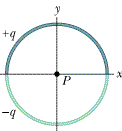# Problem: In the figure, two curved plastic rods, one of charge +q and the other of charge -q, form a circle of radius R = 8.58 cm in an xy plane. The x-axis passes through both of the connecting points, and the charge is distributed uniformly on both rods. If q = 17.2 pC, what is the magnitude of the electric field produced at P, the center of the circle?

###### FREE Expert Solution

The electric field due to a semi-circle is:

$\overline{){\mathbf{E}}{\mathbf{=}}\frac{\mathbf{2}\mathbf{k}\mathbf{q}}{\mathbf{\pi }{\mathbf{R}}^{\mathbf{2}}}}$

From the given figure, the upper part of the circle (semi-circle) is positively charged.

The electric field in this part will point radially outwards the negative y-axis.

Likewise, the bottom part of the circle is negatively charged.

90% (417 ratings)###### Problem DetailsIn the figure, two curved plastic rods, one of charge +q and the other of charge -q, form a circle of radius R = 8.58 cm in an xy plane. The x-axis passes through both of the connecting points, and the charge is distributed uniformly on both rods. If q = 17.2 pC, what is the magnitude of the electric field produced at P, the center of the circle?